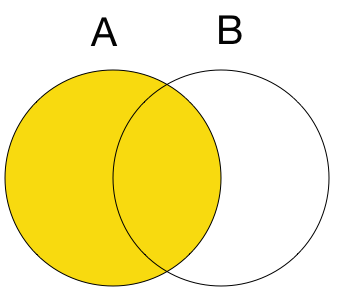# Let's Find The Other Single Event

Probability Level 1Let $A$ and $B$ be two events such that $P (A) =0.3$ and $P (A \cup B) = 0.8$. If $A$ and $B$ are independent events, find $P (B)$.

×